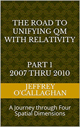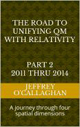The concept of space is considered to be of fundamental importance to an understanding of our physical universe. However no one has been able to define what it is physical made up of.

For example space is often defined as the boundless three-dimensional extent in which objects and events have relative position and direction and is often conceived in three linear dimensions. Yet most modern physicists include time to be part of a boundless four-dimensional continuum known as space-time defined by Einstein. However, disagreement continues between philosophers over whether it is itself an entity, a relationship between entities, or part of a conceptual framework.

One way of determining which one of these is correct would be to examine exactly how Einstein defined entities in terms of the field concepts of space-time and observations of that field.

For example he told us all objects and entities are made up of the energy contained in space-time. In other words if one accepts Einstein’s field properties of space-time one must assume that space itself is an entity. This is because due to its relativistic properties one must not only assume that a particle and all other entities can be in motion with respect to the space around it but that the space around them can be in motion while they are not. Therefore when someone views space from the perspective of a moving particle it must be consider an entity because when we view a moving particle with respect to the stationary space around it we define it as an entity. In other words according to the relativistic concepts of Einstein if one defines the energy of a quantum particle such as a proton or neutron as an entity one must also define the continuous properties of space around it as an entity.

However, one reason for the confusion involving the physical make up of space is that quantum mechanics tells us that space is granular while saying nothing about the space between them.   However, that does that does not mean that it does not exist.

Because if it is true, as Quantum mechanics suggests that space is granular then these granules must move through the volume between them so that they can interact with the next particle in the particle train. This is true no matter how small they are.  The question then becomes "What is the space between them made up of?"

Additionally the wave properties of electromagnetic energy tell us that  it must be traveling on the continuous field properties of four-dimensional space-time because wave energy can only be propagated on a continuous medium. Granted we do observe breaks in it which are associated with entities called particles.

Quantum Mechanics tells these breaks in space can be calculated using the continuous mathematical properties associated with SchrÃ¶dinger’s wave equation. Yet it assumes this wave equation only defines a probability function or an abstract property of space where a particle can be found and not a physical property of the medium it is traveling through.

Yet even this suggests Einstein’s concept of space being made up of a continuous field is accurate in a quantum environment, because the mathematical properties of SchrÃ¶dinger’s wave equation are continuous which, even though it is interpreted as being abstract is the "medium" quantum mechanics tells us supports the existence of a particle.

Additionally Einstein also tells us the energy of the curvature in space-time associated with the mass of entities including all particles extends to the ends of the universe. However this means the space between all particles and entities contains the energy component of mass or more correctly the energy/mass define by the equation E=mc^2.  Therefore if one defines an entity as that which contains energy as Einstein did one must define the space between then as an entity because it also contains energy.

This conclusion is mathematically supported by the continuous properties of SchrÃ¶dinger’s wave equation because it tells us the energy of a quanta is distributed through the entire universe in a continuous manner.

One of the difficulties philosophers and physicists have in defining space is that they do not or cannot accept the fact that the energy component of space has the physical properties of an entity as Einstein equation E=mc^2 tells us it must.  However, as shown above if one accepts Einstein and or quantum theory one must assume that it does because as was just mentioned they both tell us energy of all particles and objects is distributed throughout space.  Therefore, if one defines an entity as that which which contains energy one must also define the energy contained in empty space as an entity because Einstein showed energy is matter which is, by all definitions is an entity in a different form.

Latter Jeff

 The Road to Unifying QM with Relativity part 1 2007 thru 2010Ebook \$8.00Paper Back \$15.00 The Road to Unifying QM with Relativity part 2 2011 thru 2014Ebook \$8.00Paper Back \$16.00 The Road to Unifying QM with Relativity part 3 2015 thru 2020Ebook \$8.00Paper Back \$18.00